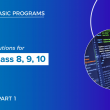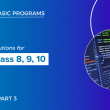# Qbasic programs solutions(part 2) for class 8, class 9 and class 10

Here, I have listed some of the qbasic programs. We also have mobile app where you can view all the qbasic programs. For better experience I highy recommend you to use our mobile app

21  Write a program to input principal, rate , time and display total amount.

``````REM PROGRAM TO DISPLAY TOTAL AMOUNT
CLS
INPUT “ENTER PRINCIPAL”; P
INPUT “ENTER TIME”; T
INPUT “ENTER RATE”;R
I = P* T * R / 100
A = P + I
PRINT “TOTAL AMOUNT=”; A
END ``````

22  Write a program to display area of square.

``````REM PROGRAM TO DISPLAY AREA OF SQUARE
CLS
INPUT “ENTER LENGTH”; L
A = L ^ 2
PRINT “AREA OF SQUARE ”; A
END
﻿``````

23  Write a program to   enter any two numbers and display the smaller one

``````REM
enter any two numbers and display the smaller one
CLS
INPUT “ENTER ANY TWO NUMBERS”; A, B
IF A < B THEN
PRINT A; “IS SMALLER”
ELSE
PRINT B; “IS SMALLER”
END IF
END ``````

24  Write a program to ask n number and print the sum of first n natural numbers.

``````REM CALCULATE SUM OF FIRST N NATURAL NUMBERS
CLS
INPUT “ENTER THE NUMBER”; N
S = N * (N + 1) / 2
PRINT “SUM OF”; N; “NUMBERS=”; S
END
﻿``````

25  Write a program to ask n number and print the sum square of first n natural numbers.

``````REM CALCULATE SUM OF SQUARE OF FIRST N NATURAL NUMBERS
CLS
INPUT “ENTER THE NUMBER”; N
S = N * (N + 1) * (2 * N + 1) / 6
PRINT “SUM OF SQUARE OF ”; N; “NUMBERS=”; S
END ``````

26  Write a program to display total surface area of hemisphere.

``````REM PROGRAM TO DISPLAY TOTAL SURFACE AREA OF HEMISPHERE.
CLS
A= 3 * 3.14 * R ^ 2
PRINT “TOTAL SURFACE AREA OF HEMISPHERE “; A
END ``````

27  Write a program to display area of circle

``````REM PROGRAM TO DISPLAY AREA OF CIRCLE
CLS
A = 3.14 * R ^ 2
PRINT “AREA OF CIRCLE ”; A
END ``````

28  Write a program to display total surface area and volume of cylinder.

``````REM PROGRAM TO DISPLAY TOTAL SURFACE AREA AND VOLUME OF CYLINDER
CLS
INPUT “ENTER HEIGHT”; H
A= 2 * 3.14 * R * (R + H)
V = 3.14 * R ^ 2 * H
PRINT “TOTAL SURFACE AREA OF CYLINDER “; A
PRINT “VOLUME OF CYLINDER ”; V
END ``````

29  Write a program to ask the user to enter the marks in any four subject and display whether the user is pass or fail.

``````CLS
INPUT "ENTER ANY FOUR SUBJECT'S MARK;"; a, b, c, d
total = a + b + c + d
avg = total / 4
IF avg > 40 THEN
PRINT "You are pass"
ELSE
PRINT "you are fail"
END IF
END``````

30  Write a program to display volume of cylinder.

``````REM PROGRAM TO DISPLAY VOLUME OF CYLINDER.
CLS
INPUT “ENTER HEIGHT”; H
V = 3.14 * R ^ 2 * H
PRINT “VOLUME OF CYLINDER ”; V
END
﻿``````

31  Write a program to display volume of hemisphere.

``````REM PROGRAM TO DISPLAY VOLUME OF HEMISPHERE.
CLS
V = (2 / 3) * 3.14 * R ^ 3
PRINT “VOLUME OF HEMISPHERE ”; V
END ``````

32  Write a program to display total surface area and volume of cube.

``````REM PROGRAM TO DISPLAY TOTAL SURFACE AREA AND VOLUME OF CUBE
CLS
INPUT “ENTER LENGTH”; L
A= 6 * L ^ 2
V = L ^ 3
PRINT “TOTAL SURFACE AREA OF CUBE “; A
PRINT “VOLUME OF CUBE ”; V
END ``````

33  Write a program to display area of triangle.

``````REM PROGRAM TO DISPLAY AREA OF TRIANGLE
CLS
INPUT “ENTER HEIGHT”; H
A = 1 / 2  * (B * H)
PRINT “AREA OF TRIANGLE “; A
END
﻿``````

34  Write a program to display volume of cube

``````REM PROGRAM TO DISPLAY VOLUME OF CUBE
CLS
INPUT “ENTER LENGTH”; L
V = L ^ 3
PRINT “VOLUME OF CUBE ”; V
END ``````

35  Write a program to display perimeter of square.

``````REM PROGRAM TO DISPLAY PERIMETER OF SQUARE
CLS
INPUT “ENTER LENGTH”; L
P = 4 * L
PRINT “PERIMETER OF SQUARE ”; P
END ``````

36  Write a program to display area of parallelogram

``````REM PROGRAM TO DISPLAY AREA OF PARALLELOGRAM
CLS
INPUT “ENTER HEIGHT”; H
A = B * H
PRINT “AREA OF PARALLELOGRAM"; A
END
﻿``````

37  Write a program to display volume of cuboid / box.

``````REM PROGRAM TO DISPLAY VOLUME OF CUBOID.
CLS
INPUT “ENTER LENGTH”; L
INPUT “ENTER HEIGHT”; H
V = L * B * H
PRINT “VOLUME OF CUBOID ”; V
END
﻿``````

38  Write a program to ask value in meter and convert into inch.

``````REM PROGRAM TO CONVERT VALUE IN METER TO INCH
CLS
INPUT”ENTER VALUE IN METER”; M
I = M * 39.37
PRINT “VALUE IN INCH=”; I
END``````

39  Write a program to display total surface area and volume of hemisphere.

``````REM PROGRAM TO DISPLAY TOTAL SURFACE AREA AND VOLUME OF HEMISPHERE.
CLS
A= 3 * 3.14 * R ^ 2
V = (2 / 3) * 3.14 * R ^ 3
PRINT “TOTAL SURFACE AREA OF HEMISPHERE “; A
PRINT “VOLUME OF HEMISPHERE ”; V
END
﻿``````

40  Write a program to display area and perimeter of square.

``````REM PROGRAM TO DISPLAY AREA AND PERIMETER OF SQUARE
CLS
INPUT “ENTER LENGTH”; L
A= L ^ 2
P = 4 * L
PRINT “AREA OF SQUARE “; A
PRINT “PERIMETER OF SQUARE ”; P
END ``````

You can view other Qbasic programs in 3rd part of this post

##### 1 comment
1.Dong Rytuba says:

Way cool! Some extremely valid points! I appreciate you penning this write-up and the rest of the site is really good.

##### Previous Post## Qbasic programs with solutions(part 1) for class 8, class 9 and class 10

##### Next Post## Qbasic programs solutions(part 3) for class 8, class 9 and class 10

##### Related Posts## QBasic Games

Introduction The world of computing has made significant progress since the early days of personal computers. Back then,…## Basic Commands in QBASIC

Welcome to the world of QBASIC programming! If you’re new to coding or just starting out, QBASIC is…## QBasic and BASIC

Introduction: Welcome to our journey through programming history! Today, we’ll explore two important programming languages from the past…